##### Question

In: Statistics and Probability

# 6) In a random sample of​ males, it was found that 25 write with their left...

6) In a random sample of​ males, it was found that 25 write with their left hands and 209 do not. In a random sample of​ females, it was found that 75 write with their left hands and 454 do not. Use a 0.01 significance level to test the claim that the rate of​ left-handedness among males is less than that among females. Complete parts​ (a) through​ (c) below.

a1) Test the claim using a hypothesis test

Consider the first sample to be the sample of males and the second sample to be the sample of females. What are the null and alternative hypotheses for the hypothesis​ test?

a2) Identify the test statistic (Round to two decimal places as needed)

a3) Identify the P Value (Round to three decimal places as needed)

a4) What is the conclusion based on the hypothesis?

The P-Value is _____(greater than/less than) the significance level of alpha = 0.01, so _______(fail to reject/reject) the null hypothesis. There is _______(sufficient/is not sufficient) evidence to support the claim that the rate of left-handedness among males is less than that among females.

b1) Test the claim by constructing an appropriate confidence interval.

The 98% confidence interval is ____ < (p1-p2) < ____ (Round to three decimal places as needed)

b2) What is the conclusion based on the confidence interval?

Because the confidence interval limits ______(include/does not include) 0, it appears that the two rates of left-handedness are ____(equal/not equal/equivalent). There is _____(sufficient/not sufficient) evidence to support the claim that the rate of left handedness among males is less than that among females.

c1) Based on the results, is the rate of left handedness among males less than the rate of left handedness among females?

a) The rate of left handedness among males does appear to be less than the rate of left handedness among females because the results are not statistically significant.

b) The rate of left handedness among males does appear to be less than the rate rate of left handedness among females because the results are statistically significant

c) The rate of left handedness among males does not appear to be less than the rate of left handedness among females

d) The results are inconclusive

## Solutions

##### Expert Solution

in sample 1 for males , we have that the sample size is n1​=234, the number of favorable cases is x1=25, so then the sample proportion is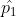=x1/n1​​=25/234​=0.1068

in sample 2 for females , we have that the sample size is n2=529, the number of favorable cases is x2=75, so then the sample proportion is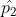=x1/n1​​=75/529​=01418

The value of the pooled proportion is computed as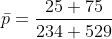= 0.1311

the given significance level is α=0.01.

a1)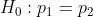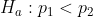a2) the test statistic (z) =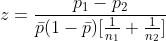= -1.32

a3) The p-value is p = 0.094

a4) The P-Value is greater than the significance level of alpha = 0.01, so fail to reject the null hypothesis. There is not sufficient evidence to support the claim that the rate of left-handedness among males is less than that among females.

b1) The 98% confidence interval is −0.094<p1​−p2​<0.024.

b2)Because the confidence interval limits include 0, it appears that the two rates of left-handedness are equal. There is not sufficient evidence to support the claim that the rate of left handedness among males is less than that among females.

c1 ) b) The rate of left handedness among males does appear to be less than the rate rate of left handedness among females because the results are statistically significant

## Related Solutions

##### 9. In a random sample of​ males, it was found that 25 write with their left...
9. In a random sample of​ males, it was found that 25 write with their left hands and 223 do not. In a random sample of​ females, it was found that 74 write with their left hands and 436 do not. Use a 0.01 significance level to test the claim that the rate of​ left-handedness among males is less than that among females. Complete parts​ (a) through​ (c) below. a. Test the claim using a hypothesis test. Consider the first...
##### In a random sample of​ males, it was found that 25 write with their left hands...
In a random sample of​ males, it was found that 25 write with their left hands and 223 do not. In a random sample of​ females, it was found that 68 write with their left hands and 442 do not. Use a 0.01 significance level to test the claim that the rate of​ left-handedness among males is less than that among females. Complete parts​ (a) through​ (c) below. (a.) Test the claim using a hypothesis test. Consider the first sample...
##### In a random sample of​ males, it was found that 26 write with their left hands...
In a random sample of​ males, it was found that 26 write with their left hands and 213 do not. In a random sample of​ females, it was found that 64 write with their left hands and 464 do not. Use a 0.01 significance level to test the claim that the rate of​ left-handedness among males is less than that among females. Complete parts​ (a) through​ (c) below. a. Test the claim using a hypothesis test. Consider the first sample...
##### In a random sample of​ males, it was found that 26 write with their left hands...
In a random sample of​ males, it was found that 26 write with their left hands and 216 do not. In a random sample of​ females, it was found that 64 write with their left hands and 455 do not. Use a 0.01 significance level to test the claim that the rate of​ left-handedness among males is less than that among females. Complete parts​ (a) through​ (c) below. a. Test the claim using a hypothesis test. b. Identify the test...
##### In a random sample of​ males, it was found that 27 write with their left hands...
In a random sample of​ males, it was found that 27 write with their left hands and 223 do not. In a random sample of​ females, it was found that 72 write with their left hands and 464 do not. Use a 0.01 significance level to test the claim that the rate of​ left-handedness among males is less than that among females. Complete parts​ (a) through​ (b) below. a) Identify the test statistic. Z= ___ b) Identify the P-value =...
##### In a random sample of​ males, it was found that 26 write with their left hands...
In a random sample of​ males, it was found that 26 write with their left hands and 217 do not. In a random sample of​ females, it was found that 61 write with their left hands and 438 do not. Use a 0.01 significance level to test the claim that the rate of​ left-handedness among males is less than that among females. Find the test statistic Z to two or more decimals and the P-value to three or more.
##### In a random sample of males, it was found that 23 write with their left hands...
In a random sample of males, it was found that 23 write with their left hands and 217 do not. In a random sample of females, it was found that 65 write with their left hands and 455 do not. We want to use a 0.01 significance level to test the claim that the rate of left-handedness among males is less than that among females. Test the claim using a hypothesis test. Group of answer choices A.P value=0.0926; fail to...
##### In a random sample of​ males, it was found that 28 write with their left hands...
In a random sample of​ males, it was found that 28 write with their left hands and 225 do not. In a random sample of​ females, it was found that 62 write with their left hands and 441 do not. Use a 0.05 significance level to test the claim that the rate of​ left-handedness among males is less than that among females. Complete parts​ (a) through​ (c) below.
##### In a random sample of​ males, it was found that 1919 write with their left hands...
In a random sample of​ males, it was found that 1919 write with their left hands and 215215 do not. In a random sample of​ females, it was found that 6969 write with their left hands and 453453 do not. Use a 0.050.05 significance level to test the claim that the rate of​ left-handedness among males is less than that among females. Complete parts​ (a) through​ (c) below. a. Test the claim using a hypothesis test. Consider the first sample...
##### In a random sample of​ males, it was found that 21 write with their left hands...
In a random sample of​ males, it was found that 21 write with their left hands and 219 do not. In a random sample of​ females, it was found that 64 write with their left hands and 437 do not. Use a 0.01 significance level to test the claim that the rate of​ left-handedness among males is less than that among females. Complete parts​ (a) through​ (c) below. a) identify the test statistic b) identify p-value c) the 90% confidence...